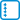## Dice roll

Find out the probability that when we roll 12 dice, we get exactly 30 pips together.

• #### Solution

The generating function for the probabilities of the sums of thrown pips is

$$\displaystyle P(x)= \frac1{6^{12}}(x+x^2+…+x^6)^{12}= \frac{x^{12}}{6^{12}}(1+x+x^2+…+x^5)^{12}= \frac{x^{12}}{6^{12}}\left(\frac{1-x^6}{1-x}\right)^{12}$$

We develop the denominator of the last term according to the generalized binomial theorem as:

$$\displaystyle (1-x)^{-12}= \sum_{k=0}^\infty\binom{-12}{k}(-x)^k$$

Coefficient by $$x^{30}$$ in $$P(x)$$ is $$\frac1{6^{12}}$$ of the coefficient of $$x^{18}$$ in the product $$\displaystyle (1-x^6)^{12}\sum_{k=0}^\infty\binom{-12}{k}(-x)^k$$.

From the first factor we select members with an exponent of at most 18 and we supplement them with members from the second factor with exponents equal to the complement up to 18. We now get:

$$\displaystyle (1-x^6)^{12}\sum_{k=0}^\infty\binom{-12}{k}(-x)^k= \left(\binom{12}{0}(-x^6)^0+\binom{12}{1}(-x^6)^1+\binom{12}{2}(-x^6)^{2} +\binom{12}{3}(-x^6)^{3}+\cdots\right)\\ \left( \binom{-12}{0}(-x)^{0}+\cdots+ \binom{-12}{6}(-x)^{6}+\cdots+ \binom{-12}{12}(-x)^{12}+\cdots+ \binom{-12}{18}(-x)^{18}+\cdots \right) =\\ \cdots+ \left( \binom{12}{0}\binom{-12}{18}- \binom{12}{1}\binom{-12}{12}+ \binom{12}{2}\binom{-12}{6}- \binom{12}{3}\binom{-12}{0} \right)x^{18}+\cdots$$

After an adjustment $$\binom{-12}{i}=\binom{i+12-1}{i}$$ and further simplification we get the result.

Note: the same result would be obtained with approximately as complex calculation using the principle of inclusion and exclusion.

The probability of throwing 30 pips on 12 dice is $$\displaystyle \frac1{6^{12}}\left( \binom{29}{18}- 12\binom{23}{12}+ \binom{12}{2}\binom{17}{6}- \binom{12}{3} \right)=\frac{9\,594\,475}{1\,088\,391\,168}\doteq 0.00881528193363657$$, so almost 9 per mille.